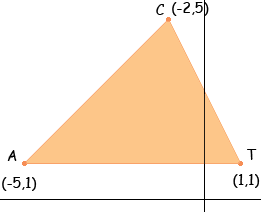i am a 7thgrader student....i need help on this!!!!!! Triangle CAT has vertices located at C(-2,5), A(-5,1), T(1,1) Translate triangle CAT 4 units right and magnify the triangle by 3. List the new coordinates and explain the process of computing the new coordinates. Graph triangle CAT and the new triangle form part A on the same coordinate plane.... Will the area of the new triangle be 3 times as large as the original?? Explain why or why not Hi, Start with a diagram.If you translate the triangle 4 units to the right then T will move from (1,1) to T (5,1), that is you add 4 to its X-coordinate. Perform the same operation on C and A. This gives you a second triangle CAT. Draw it on the same diagram. The instruction " magnify the triangle by 3" means you want a new triangle that has sides three times as long as the sides of CAT. For example the length of the side from A to T is 6 units and hence the magnified triangle with have this side expanded to a length of 63 = 18 units. What if you multiply all the coordinates of CAT by 3? Does this give a triangle of the correct size? Try it and draw the triangle. Check its side lengths by either measuring or using Pythagoras Theorem. Draw CAT and this new translated and magnified triangle on the same diagram. Can you fit CAT inside the new triangle more than 3 times? Less than 3 times? Exactly 3 times? Penny